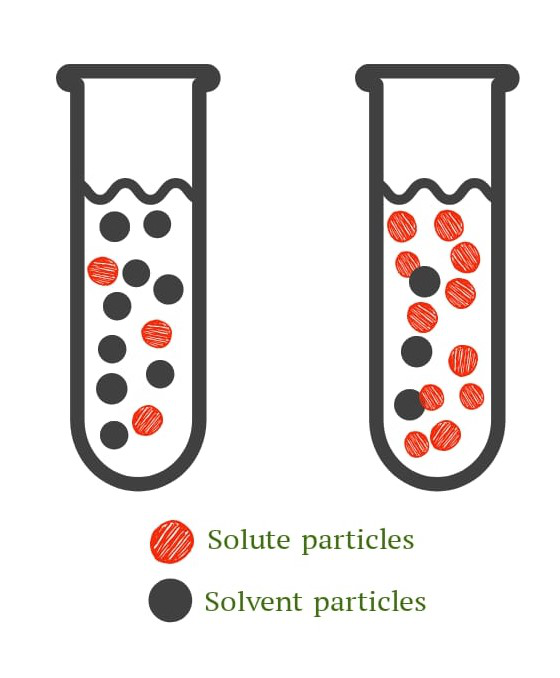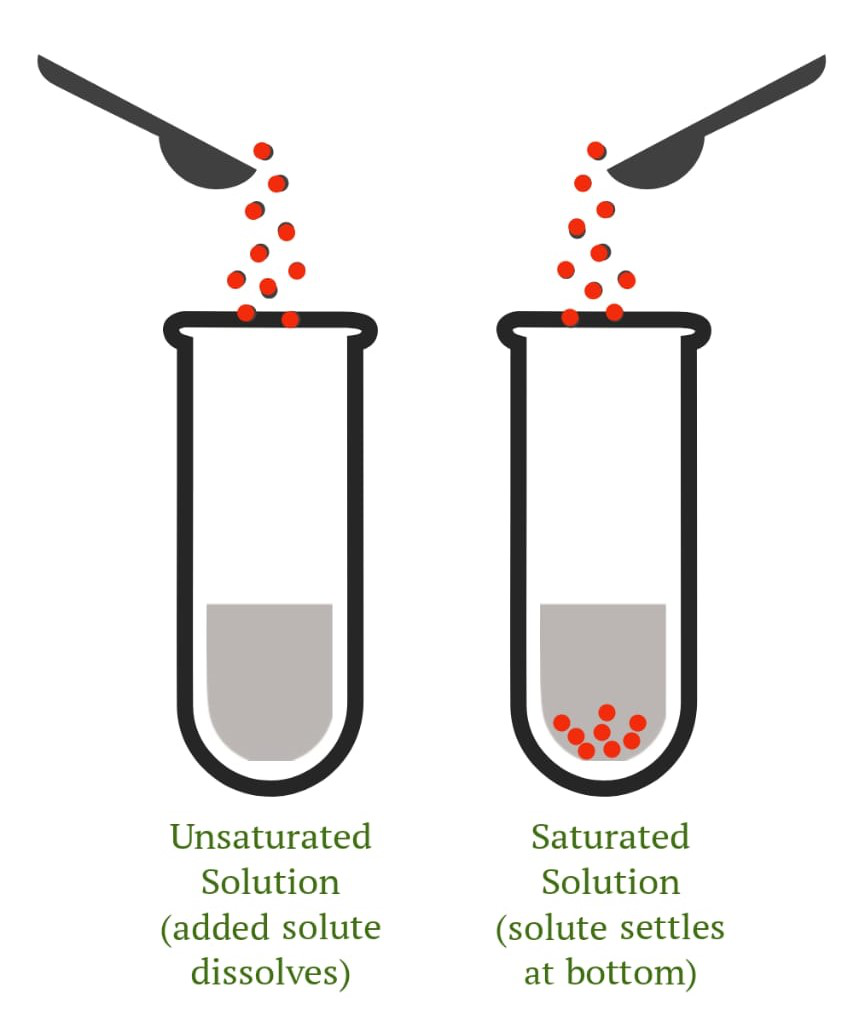# Concentration of a Solution

• Last Updated : 21 Sep, 2021

Concentration is a frequently used term in chemistry and other relevant fields. It is a means of determining how much of one substance is mixed with another substance. This can apply to any type of chemical combination, although it is most commonly used in the context of solutions, where it refers to the quantity of solute dissolved in a solvent. Concentration, can be expressed in both qualitative (informal) or quantitative (numerically) terms.

The concentration of a solution:

• determines the amount of solute dissolved in a unit amount of solvent,
• can be represented qualitatively and quantitatively, and
• is a macroscopic property  (The characteristics of matter in bulk are referred to as macroscopic properties)

### Qualitative Expressions of Concentration

To qualitatively express concentration, a solution can be classified as a, dilute solution or a concentrated solution.

1. Dilute Solution : A dilute solution contains a smaller proportion of solute as compared to the proportion of solvent.
2. Concentrated Solution : A dilute solution contains a much greater proportion of solute as compared to the proportion of solvent.Figure 1. Dilute (left) and Concentrated (right) solutions

### Semi-Qualitative Expressions of Concentration

To semi-qualitatively express concentration, a solution can be classified as an, saturated solution or a unsaturated solution.

1. Saturated Solution : A solvent has the ability to dissolve certain types of solutes. A saturated solution is the greatest quantity of solute that may be dissolved in a solvent at a given temperature. When a solution reaches saturation, it can no longer dissolve any more solute. Undissolved chemicals settle to the bottom. The saturation point is determined as point at which the no more solute can be dissolved in the solvent.
2. Unsaturated Solution : An unsaturated solution is one that contains less solute than the maximum value, that is, before the solution reaches the saturation level. There are no residual substances at the bottom, indicating that all of the solute has been dissolved in the solvent. An unsaturated solution is a chemical solution in which the solute concentration is less than the corresponding equilibrium solubility.Figure 2. Unsaturated (left) and Saturated (right) solutions

From the above terms we can define solubility :

Solubility is defined as the greatest amount of solute that may dissolve in a certain quantity of solvent at a given temperature.

A solution is a liquid that is a homogeneous combination of one or more solutes and a solvent. A typical example of a solution is, sugar cubes added to a cup of tea or coffee. Here, solubility is the characteristic that allows sugar molecules to dissolve.

As a result, the term solubility may be defined as a substance’s (solute’s) ability to dissolve in a particular solvent.

### Quantitative Expressions of Concentration

To quantitatively express concentration, we use the following terms:

• Mass by Mass percentage of a solution
• Mass by Volume percentage of a solution

Qualitative expressions of concentration are relative terms, which do not provide the exact concentration of the solution. To characterize the concentrations of various solutions around us in an accurate and precise manner, we require quantitative expressions of concentration.

Generally, concentration is represented in both ways:

• Concentration = Quantity of Solute / Quantity of Solution

or

• Concentration = Quantity of Solute / Quantity of Solvent

Mass by Mass percentage (w/w%): It describes the amount of solute (grams) present in 100 gm of the solution.

Here, both the mass of solute and the mass of solution will be in grams.

Mass by Mass % = (Mass of Solute / Mass of Solution) × 100

Mass by Mass percentage has no unit.

Mass by Volume percentage (w/v%): It describes the amount of solute (grams) present in 100 mL of solution.

Mass by Volume % = (Mass of Solute / Volume of Solution) × 100

Here, the mass of solute will be in grams and the volume of solution will be in mL.

Mass by Volume percentage has the unit g/mL.

### Sample Questions

Question 1: 15 g of common salt is dissolved in 400 g of water. Calculate the concentration of the solution by expressing it in Mass by Mass percentage (w/w%).

Given that,

Mass of solute (common salt) = 15 g    …(1)

Mass of Solvent (water) = 400 g    …(2)

It is known that,

Mass of Solution = Mass of Solute + Mass of Solvent    …(3)

So,

Substituting (1) and (2) in (3), we obtain the following,

Mass of Solution = 15 g + 400 g = 415 g    …(4)

From Figure 4, we know

Mass by Mass Percentage = ( Mass of Solute / Mass of Solution ) × 100    …(5)

Substituting (1) and (4) in (5), we obtain the following,

Mass by Mass Percentage = ( 15 g / 415 g ) × 100 = ( 0.0361 ) × 100 = 3.61

( w / w % ) = 3.61

Question 2: 15 g of common salt is dissolved in a solution of 300 mL, calculate the Mass by Volume percentage (w/v%).

Given that,

Mass of solute (common salt) = 15 g     …(1)

Mass of Solution (salt solution) = 300 mL    …(2)

From Figure 5, we know

Mass by Volume Percentage = ( Mass of Solute / Volume of Solution ) × 100     …(3)

Substituting (1) and (2) in (3), we obtain the following,

Mass by Volume Percentage = ( 15 g / 300 mL ) × 100 = ( 0.05 ) × 100 = 5 g/mL

( w / v % ) = 5 g/mL

Question 3: Define Solubility.

Solubility is defined as the greatest amount of solute that may dissolve in a certain quantity of solvent at a given temperature.

Question 4: Richard dissolved 70 g of sugar in 750 mL of sugar solution. Calculate the Mass by Volume percentage (w/v%).

Given that,

Mass of solute (common salt) = 70 g     …(1)

Mass of Solution (salt solution) = 750 mL     …(2)

From Figure 5, we know

Mass by Volume Percentage = ( Mass of Solute / Volume of Solution ) × 100     …(3)

Substituting (1) and (2) in (3), we obtain the following,

Mass by Volume Percentage = ( 70 g / 750 mL ) * 100 = ( 0.933 ) × 100 = 93.3 g/mL

( w / v % ) = 93.3 g/mL

Question 5: What is a dilute solution?

A dilute solution is solution which contains a smaller proportion of solute as compared to the proportion of solvent.

Question 6: Is Mass by Mass % dependent on temperature?

No, mass by mass % is not dependent on temperature as mass is no effected by temperature changes.

Question 7: Is Mass by Volume % dependent on temperature?